# Linear Regression for College Tuition in R

Required files can be downlowded here.

The following exercises aim to use linear regression tools in R to investigate whether a linear relationship exists between tuition and other variables. The dataset for these tasks is called tuition.csv. The dataset has 1283 records of information about school tuition. The data table (d0) are structured as the table below.

VARIABLESDESCRIPTIONDATA TYPE
tuitionCollege tuition (“out-of-state” rate)continuous
pcttop25Percent of new students from the top 25% of high school classcontinuous
sf_ratioStudent to faculty ratiocontinuous
fac_compAverage faculty compensationcontinuous
accrateFraction of applicants accepted for admissioncontinuous
pct_phdPercent of faculty with Ph.D.’scontinuous
fulltimePercent of undergraduates who are full time studentscontinuous
alumniPercent of alumni who donatecontinuous
num_enrlNumber of new students enrolledcontinuous
public.privateIs the college a public or private institution? public=0, private=1discrete

a) Check the names and data types of variables (columns) in R. And compare the ones in above table. Take actions to make sure that there is no problem with the data types: Integer or Numeric for continuous variables; Factor for discrete variables.

d0 <- read.csv("tuition.csv")
d0$public.private <- factor(d0$public.private, levels = c(0, 1),
labels = c("public", "private"))
str(d0)
## 'data.frame':    1283 obs. of  11 variables:
##  $tuition : int 3400 5600 4440 3000 6300 2600 4104 11660 2970 8080 ... ##$ pcttop25      : int  NA 27 78 NA 57 6 46 88 40 47 ...
##  $sf_ratio : num 14.3 32.8 18.9 18.7 16.7 16.5 25.3 14 19.4 11.4 ... ##$ fac_comp      : int  42300 NA 47700 NA 54600 NA 54800 52300 50300 36600 ...
##  $accrate : num 0.682 0.928 0.66 0.705 0.9 1 0.735 0.73 0.646 0.855 ... ##$ graduat       : int  40 55 51 15 69 36 50 72 76 44 ...
##  $pct_phd : int 53 52 72 48 85 64 94 74 62 63 ... ##$ fulltime      : num  92.8 70.3 87.8 90.9 90.5 ...
##  $alumni : int NA NA 8 NA 18 NA 3 34 5 9 ... ##$ num_enrl      : int  984 179 570 1278 3070 644 1686 287 625 127 ...
##  $public.private: Factor w/ 2 levels "public","private": 1 2 1 1 1 1 1 2 1 2 ... b) Missing data appears to be a problem with this data set. Prepare a copy of the data set (d1), where the missing values are each replaced with their column means. Try to use for loop in R for this question. d1 <- d0 for (i in 1:(ncol(d1)-1)) { d1[which(is.na(d0[, i])), i] <- mean(d1[, i], na.rm = TRUE) } c) Report on how this imputation has affected the summary statistics between d0 and d1. What do you think of this method of dealing with missing values? Can you suggest a better method? summary(d0) ## tuition pcttop25 sf_ratio fac_comp ## Min. : 1044 Min. : 6.00 Min. : 2.30 Min. : 26500 ## 1st Qu.: 6114 1st Qu.: 37.00 1st Qu.:11.80 1st Qu.: 43600 ## Median : 8670 Median : 50.00 Median :14.30 Median : 50900 ## Mean : 9284 Mean : 52.28 Mean :14.87 Mean : 52680 ## 3rd Qu.:11675 3rd Qu.: 65.00 3rd Qu.:17.60 3rd Qu.: 60100 ## Max. :25750 Max. :100.00 Max. :91.80 Max. :107500 ## NA's :196 NA's :2 NA's :162 ## accrate graduat pct_phd fulltime ## Min. :0.1540 Min. : 8.00 Min. : 8.00 Min. :11.43 ## 1st Qu.:0.6837 1st Qu.: 47.00 1st Qu.: 57.00 1st Qu.:68.59 ## Median :0.7840 Median : 60.00 Median : 71.00 Median :83.42 ## Mean :0.7581 Mean : 60.42 Mean : 68.72 Mean :78.79 ## 3rd Qu.:0.8610 3rd Qu.: 74.00 3rd Qu.: 82.00 3rd Qu.:91.88 ## Max. :1.0000 Max. :118.00 Max. :103.00 Max. :99.94 ## NA's :11 NA's :95 NA's :31 NA's :27 ## alumni num_enrl public.private ## Min. : 0.00 Min. : 18.0 public :457 ## 1st Qu.:11.00 1st Qu.: 234.5 private:826 ## Median :19.00 Median : 446.5 ## Mean :20.92 Mean : 782.4 ## 3rd Qu.:29.00 3rd Qu.: 984.2 ## Max. :81.00 Max. :7425.0 ## NA's :213 NA's :3 summary(d1) ## tuition pcttop25 sf_ratio fac_comp ## Min. : 1044 Min. : 6.00 Min. : 2.30 Min. : 26500 ## 1st Qu.: 6114 1st Qu.: 39.00 1st Qu.:11.80 1st Qu.: 44700 ## Median : 8670 Median : 52.28 Median :14.30 Median : 52680 ## Mean : 9284 Mean : 52.28 Mean :14.87 Mean : 52680 ## 3rd Qu.:11675 3rd Qu.: 63.00 3rd Qu.:17.55 3rd Qu.: 58500 ## Max. :25750 Max. :100.00 Max. :91.80 Max. :107500 ## accrate graduat pct_phd fulltime ## Min. :0.1540 Min. : 8.00 Min. : 8.00 Min. :11.43 ## 1st Qu.:0.6850 1st Qu.: 48.00 1st Qu.: 57.00 1st Qu.:68.91 ## Median :0.7820 Median : 60.42 Median : 70.00 Median :82.89 ## Mean :0.7581 Mean : 60.42 Mean : 68.72 Mean :78.79 ## 3rd Qu.:0.8605 3rd Qu.: 72.00 3rd Qu.: 82.00 3rd Qu.:91.59 ## Max. :1.0000 Max. :118.00 Max. :103.00 Max. :99.94 ## alumni num_enrl public.private ## Min. : 0.00 Min. : 18.0 public :457 ## 1st Qu.:12.00 1st Qu.: 235.5 private:826 ## Median :20.92 Median : 449.0 ## Mean :20.92 Mean : 782.4 ## 3rd Qu.:26.00 3rd Qu.: 982.0 ## Max. :81.00 Max. :7425.0 This method has changed the median values for most of the variables, however it did not change the mean. I think this method of dealing with missing values is quite good, however it can lead to biased estimators. I cannot suggest a better method. ## Task 2. Relationships between Variables a) Provide a scatter plot describing the relationship of each variable with tuition. Examine the plots and rank them in descending order according to the correlation between each variable and tuition. Ranked scatter plots: par(mfrow = c(1, 2)) plot(tuition ~ graduat, data = d1, las = 1) plot(tuition ~ alumni, data = d1, las = 1)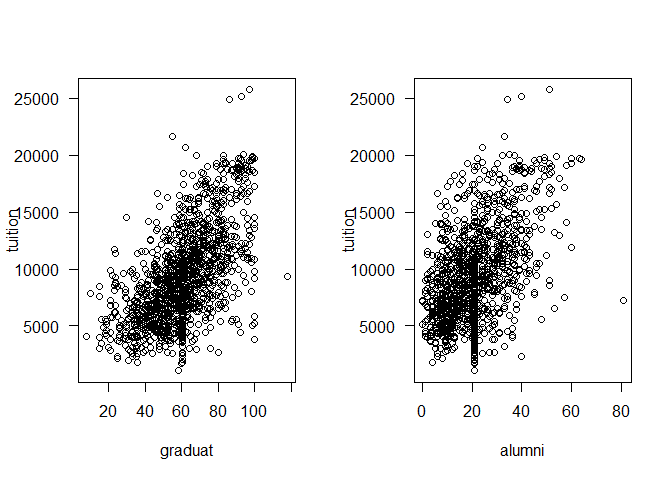plot(tuition ~ pcttop25, data = d1, las = 1) plot(tuition ~ fac_comp, data = d1, las = 1)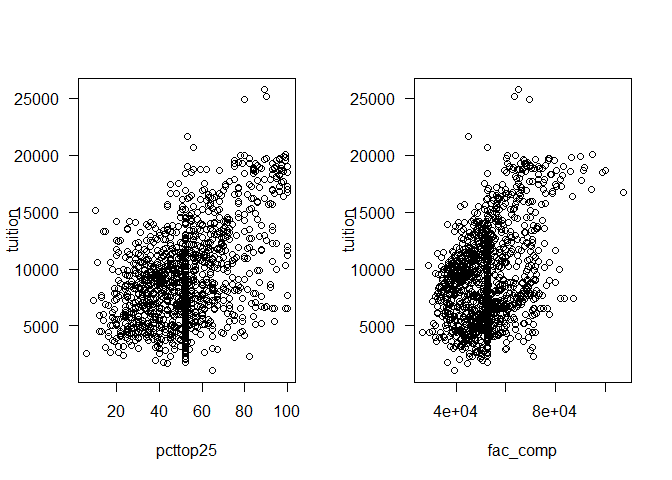plot(tuition ~ pct_phd, data = d1, las = 1) plot(tuition ~ fulltime, data = d1, las = 1)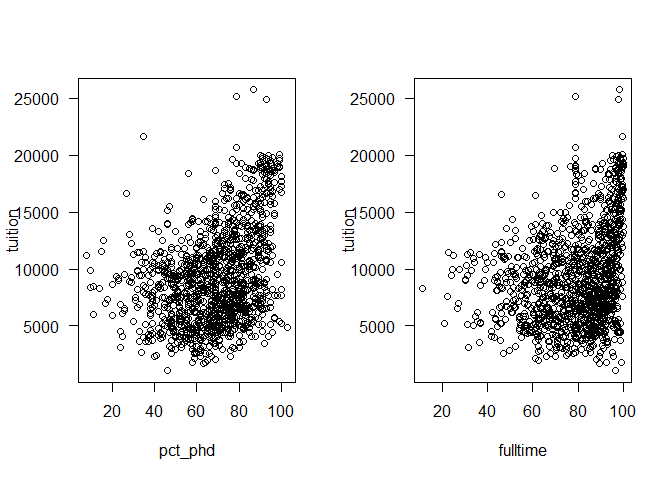plot(tuition ~ num_enrl, data = d1, las = 1) plot(tuition ~ accrate, data = d1, las = 1)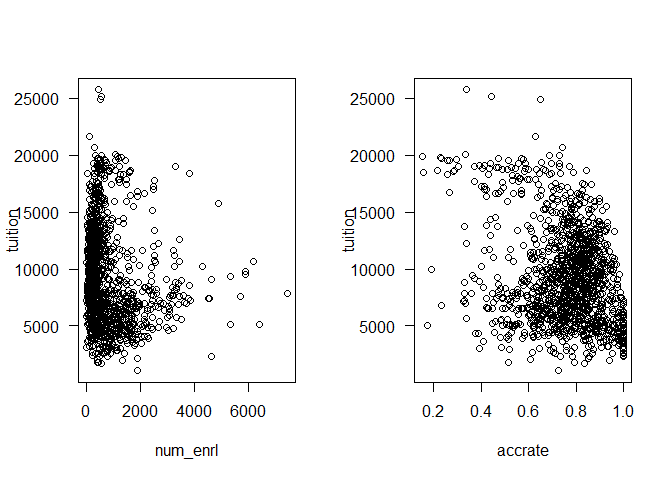plot(tuition ~ sf_ratio, data = d1, las = 1) par(mfrow = c(1, 1))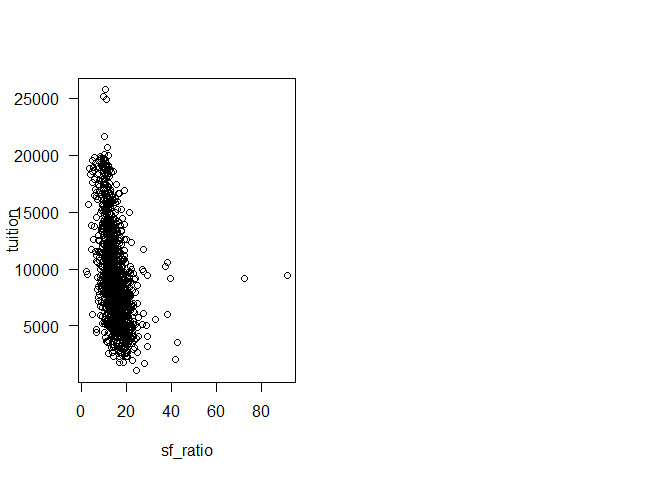b) Calculate the correlation values for each pair of relationship and validate your ranking. cor_matrix <- cor(d1[, -11]) sort(cor_matrix[, 1], decreasing = TRUE) ## tuition graduat alumni pcttop25 fac_comp pct_phd fulltime ## 1.0000000 0.6040003 0.4995750 0.4726536 0.3937655 0.3739891 0.2584426 ## num_enrl accrate sf_ratio ## -0.1396856 -0.2730378 -0.4424179 ## Task 3. Model Building a) Fit a linear regression model (L1) using all variables and data. L1 <- lm(tuition ~ ., data = d0) summary(L1) ## ## Call: ## lm(formula = tuition ~ ., data = d0) ## ## Residuals: ## Min 1Q Median 3Q Max ## -9085.9 -1257.7 -18.3 1256.7 11017.4 ## ## Coefficients: ## Estimate Std. Error t value Pr(>|t|) ## (Intercept) -2.542e+03 9.887e+02 -2.571 0.010328 * ## pcttop25 -3.048e-01 5.426e+00 -0.056 0.955208 ## sf_ratio -1.749e+02 2.319e+01 -7.544 1.25e-13 *** ## fac_comp 1.288e-01 1.043e-02 12.340 < 2e-16 *** ## accrate 6.292e+00 6.357e+02 0.010 0.992105 ## graduat 2.700e+01 5.651e+00 4.778 2.11e-06 *** ## pct_phd 3.136e+01 6.861e+00 4.572 5.61e-06 *** ## fulltime 1.259e+01 5.201e+00 2.420 0.015730 * ## alumni 4.483e+01 7.663e+00 5.850 7.17e-09 *** ## num_enrl -3.907e-01 1.120e-01 -3.490 0.000509 *** ## public.privateprivate 3.952e+03 2.487e+02 15.892 < 2e-16 *** ## --- ## Signif. codes: 0 '***' 0.001 '**' 0.01 '*' 0.05 '.' 0.1 ' ' 1 ## ## Residual standard error: 2118 on 793 degrees of freedom ## (479 observations deleted due to missingness) ## Multiple R-squared: 0.7403, Adjusted R-squared: 0.737 ## F-statistic: 226 on 10 and 793 DF, p-value: < 2.2e-16 b) Write out the estimated regression equation in mathematic formulation. $$\widehat{tuition}$$ = -2541.86 - 0.30pcttop25 - 174.91sf_ratio + 0.13fac_comp + 6.29accrate + 27.00graduat + 31.36pct_phd + 12.59fulltime + 44.83alumni - 0.39num_enrl + 3951.67public.private c) Report RMSE, R-squared, and adjusted R-squared. RMSE <- sqrt(mean(L1$residuals^2))
RMSE
##  2103.585

RMSE = 2103.585, R-squared = 0.7403, adjusted R-squared = 0.737.

a) Investigate whether the regression assumptions hold. These assumptions are linearity, normality, and constant variance.

par(mfrow = c(2, 2))
plot(L1)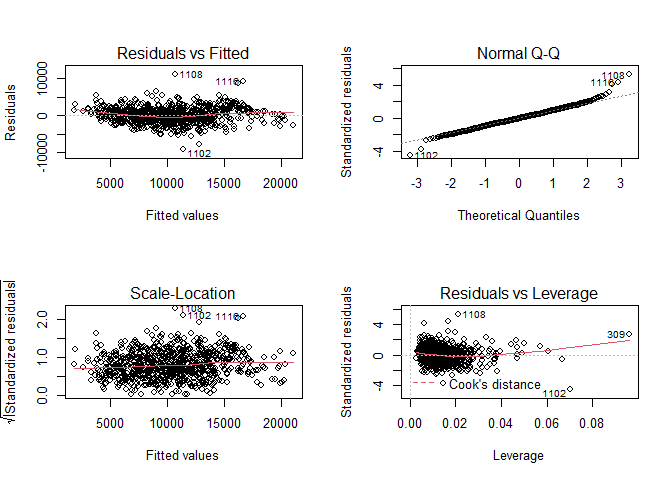par(mfrow = c(1, 1))

Linearity: The Residuals vs Fitted plot shows equally spread residuals around a horizontal line without distinct patterns, suggesting that the linearity assumption is satisfied.

Normality: The Normal Q-Q plot shows the points following the straight line quite well, suggesting that the normality assumption is satisfied,

Constant variance: The Scale-Location plot shows a horizontal line with equally (randomly) spread points, suggesting that the homoscedasticity (constant variance) assumption is satisfied.

b) Explain clearly and completely the meaning and interpretation of the regression coefficient for faculty compensation.

Holding other predictors in the model constant, every one unit increase in faculty compensation results in 0.13 increase in tuition.

c) Explain clearly and completely the meaning of the coincident for public.private.

Holding other predictors in the model constant, the tuition for private schools is about 3951.67 higher than the tuition for public schools.

a) Rebuild a linear regression model (L2) by removing some variables that you think are insignificant.

L2 <- lm(tuition ~ sf_ratio + fac_comp + graduat + pct_phd + fulltime + alumni +
num_enrl + public.private, data = d0)
summary(L2)
##
## Call:
## lm(formula = tuition ~ sf_ratio + fac_comp + graduat + pct_phd +
##     fulltime + alumni + num_enrl + public.private, data = d0)
##
## Residuals:
##     Min      1Q  Median      3Q     Max
## -9350.2 -1236.5     7.6  1226.8 10838.1
##
## Coefficients:
##                         Estimate Std. Error t value Pr(>|t|)
## (Intercept)           -2.622e+03  6.734e+02  -3.894 0.000106 ***
## sf_ratio              -1.691e+02  2.176e+01  -7.771 2.18e-14 ***
## fac_comp               1.320e-01  9.021e-03  14.629  < 2e-16 ***
## graduat                2.433e+01  5.172e+00   4.705 2.95e-06 ***
## pct_phd                2.727e+01  6.177e+00   4.415 1.14e-05 ***
## fulltime               1.582e+01  4.780e+00   3.310 0.000971 ***
## alumni                 4.629e+01  7.180e+00   6.447 1.89e-10 ***
## num_enrl              -3.811e-01  1.041e-01  -3.660 0.000268 ***
## public.privateprivate  3.914e+03  2.322e+02  16.855  < 2e-16 ***
## ---
## Signif. codes:  0 '***' 0.001 '**' 0.01 '*' 0.05 '.' 0.1 ' ' 1
##
## Residual standard error: 2092 on 876 degrees of freedom
##   (398 observations deleted due to missingness)
## Multiple R-squared:  0.7491, Adjusted R-squared:  0.7468
## F-statistic: 326.9 on 8 and 876 DF,  p-value: < 2.2e-16

b) Construct a table to compare L1 and L2 models, variables included, R-squared, and pvalue of each variable. Which model do you prefer and why?

library(stargazer)
stargazer(L1, L2, type = "text", column.labels = c("L1", "L2"), no.space = TRUE)
##
## ========================================================================
##                                      Dependent variable:
##                       --------------------------------------------------
##                                            tuition
##                                  L1                        L2
##                                  (1)                      (2)
## ------------------------------------------------------------------------
## pcttop25                       -0.305
##                                (5.426)
## sf_ratio                     -174.906***              -169.062***
##                               (23.186)                  (21.756)
## fac_comp                      0.129***                  0.132***
##                                (0.010)                  (0.009)
## accrate                         6.292
##                               (635.673)
##                                (5.651)                  (5.172)
## pct_phd                       31.364***                27.269***
##                                (6.861)                  (6.177)
## fulltime                      12.588**                 15.821***
##                                (5.201)                  (4.780)
## alumni                        44.829***                46.287***
##                                (7.663)                  (7.180)
## num_enrl                      -0.391***                -0.381***
##                                (0.112)                  (0.104)
## public.privateprivate       3,951.672***              3,914.068***
##                               (248.658)                (232.222)
## Constant                    -2,541.856**             -2,621.899***
##                               (988.745)                (673.357)
## ------------------------------------------------------------------------
## Observations                     804                      885
## R2                              0.740                    0.749
## Residual Std. Error     2,118.124 (df = 793)      2,092.359 (df = 876)
## F Statistic           226.003*** (df = 10; 793) 326.915*** (df = 8; 876)
## ========================================================================
## Note:                                        *p<0.1; **p<0.05; ***p<0.01

I prefer the L2 model because it contains only significant variables and has higher adjusted R-squared value.

## Task 6. Model Building with Data Imputation

a) Re-do task 3, by using data set d1 where the missing values are each replaced with their column means and build the linear regression model L3.

L3 <- lm(tuition ~ ., data = d1)
summary(L3)
##
## Call:
## lm(formula = tuition ~ ., data = d1)
##
## Residuals:
##     Min      1Q  Median      3Q     Max
## -9367.0 -1386.0    76.3  1402.3 12179.7
##
## Coefficients:
##                         Estimate Std. Error t value Pr(>|t|)
## (Intercept)           -4.735e+03  7.809e+02  -6.064 1.75e-09 ***
## pcttop25               9.382e+00  4.720e+00   1.988    0.047 *
## sf_ratio              -1.011e+02  1.424e+01  -7.100 2.07e-12 ***
## fac_comp               1.095e-01  8.490e-03  12.893  < 2e-16 ***
## accrate                2.554e+02  5.041e+02   0.507    0.613
## graduat                4.209e+01  4.679e+00   8.995  < 2e-16 ***
## pct_phd                3.920e+01  4.994e+00   7.849 8.83e-15 ***
## fulltime               7.704e+00  4.411e+00   1.747    0.081 .
## alumni                 4.193e+01  6.840e+00   6.130 1.17e-09 ***
## num_enrl              -1.939e-01  9.969e-02  -1.945    0.052 .
## public.privateprivate  3.888e+03  1.954e+02  19.901  < 2e-16 ***
## ---
## Signif. codes:  0 '***' 0.001 '**' 0.01 '*' 0.05 '.' 0.1 ' ' 1
##
## Residual standard error: 2363 on 1272 degrees of freedom
## Multiple R-squared:  0.6827, Adjusted R-squared:  0.6802
## F-statistic: 273.7 on 10 and 1272 DF,  p-value: < 2.2e-16

$$\widehat{tuition}$$ = -4735.07 + 9.38pcttop25 - 101.11sf_ratio + 0.11fac_comp + 255.37accrate + 42.09graduat + 39.20pct_phd + 7.70fulltime + 41.93alumni - 0.19num_enrl + 3888.06public.private

RMSE <- sqrt(mean(L3$residuals^2)) RMSE ##  2352.389 RMSE = 2352.389, R-squared = 0.6827, adjusted R-squared = 0.6802. b) Add the results of L3 to the previous table, showing variables included, R-squared, and p-value of each variable. Which model do you find more credible and why? stargazer(L1, L2, L3, type = "text", column.labels = c("L1", "L2", "L3"), no.space = TRUE) ## ## =================================================================================================== ## Dependent variable: ## ----------------------------------------------------------------------------- ## tuition ## L1 L2 L3 ## (1) (2) (3) ## --------------------------------------------------------------------------------------------------- ## pcttop25 -0.305 9.382** ## (5.426) (4.720) ## sf_ratio -174.906*** -169.062*** -101.112*** ## (23.186) (21.756) (14.242) ## fac_comp 0.129*** 0.132*** 0.109*** ## (0.010) (0.009) (0.008) ## accrate 6.292 255.371 ## (635.673) (504.103) ## graduat 27.000*** 24.334*** 42.087*** ## (5.651) (5.172) (4.679) ## pct_phd 31.364*** 27.269*** 39.201*** ## (6.861) (6.177) (4.994) ## fulltime 12.588** 15.821*** 7.704* ## (5.201) (4.780) (4.411) ## alumni 44.829*** 46.287*** 41.927*** ## (7.663) (7.180) (6.840) ## num_enrl -0.391*** -0.381*** -0.194* ## (0.112) (0.104) (0.100) ## public.privateprivate 3,951.672*** 3,914.068*** 3,888.064*** ## (248.658) (232.222) (195.374) ## Constant -2,541.856** -2,621.899*** -4,735.070*** ## (988.745) (673.357) (780.879) ## --------------------------------------------------------------------------------------------------- ## Observations 804 885 1,283 ## R2 0.740 0.749 0.683 ## Adjusted R2 0.737 0.747 0.680 ## Residual Std. Error 2,118.124 (df = 793) 2,092.359 (df = 876) 2,362.538 (df = 1272) ## F Statistic 226.003*** (df = 10; 793) 326.915*** (df = 8; 876) 273.723*** (df = 10; 1272) ## =================================================================================================== ## Note: *p<0.1; **p<0.05; ***p<0.01 I think model L2 gives more credible results because it has the largest adjusted R-squared and all variables are statistically significant. ## Task 7. Regression Model Error a) Split the original dataset into two parts, training and testing. Randomly select 70% rows of data as training part and the rest 30% as test part, using sample() R function. set.seed(123) trainRows <- sample(nrow(d0), 0.7 * nrow(d0)) mdataTrain <- d0[trainRows,] mdataTest <- d0[-trainRows,] b) Re-do task 3 by using training data only, and get a LR model (L4). L4 <- lm(tuition ~ ., data = mdataTrain) summary(L4) ## ## Call: ## lm(formula = tuition ~ ., data = mdataTrain) ## ## Residuals: ## Min 1Q Median 3Q Max ## -8964.3 -1305.9 -17.7 1245.1 10859.8 ## ## Coefficients: ## Estimate Std. Error t value Pr(>|t|) ## (Intercept) -1.851e+03 1.157e+03 -1.599 0.110380 ## pcttop25 -5.111e+00 6.462e+00 -0.791 0.429376 ## sf_ratio -1.676e+02 2.793e+01 -6.000 3.54e-09 *** ## fac_comp 1.298e-01 1.231e-02 10.545 < 2e-16 *** ## accrate -5.721e+02 7.621e+02 -0.751 0.453195 ## graduat 2.605e+01 6.695e+00 3.891 0.000112 *** ## pct_phd 3.090e+01 8.143e+00 3.795 0.000164 *** ## fulltime 1.250e+01 6.280e+00 1.991 0.046980 * ## alumni 4.513e+01 9.214e+00 4.898 1.27e-06 *** ## num_enrl -4.325e-01 1.339e-01 -3.229 0.001315 ** ## public.privateprivate 3.989e+03 2.963e+02 13.466 < 2e-16 *** ## --- ## Signif. codes: 0 '***' 0.001 '**' 0.01 '*' 0.05 '.' 0.1 ' ' 1 ## ## Residual standard error: 2156 on 559 degrees of freedom ## (328 observations deleted due to missingness) ## Multiple R-squared: 0.7363, Adjusted R-squared: 0.7316 ## F-statistic: 156.1 on 10 and 559 DF, p-value: < 2.2e-16 $$\widehat{tuition}$$ = -1850.72 - 5.11pcttop25 - 167.56sf_ratio + 0.13fac_comp - 572.06accrate + 26.05graduat + 30.90pct_phd + 12.50fulltime + 45.13alumni - 0.43num_enrl + 3989.36public.private RMSE <- sqrt(mean(L4$residuals^2))
RMSE
##  2135.556

RMSE = 2135.556, R-squared = 0.7363, adjusted R-squared = 0.7316.

c) Apply L4 for training dataset, calculate the regression performance indicators, include the Sum of Square Errors (SSE), the Mean Squared Error (MSE), and the Mean Absolute Percentage Error (MAPE).

SSE <- sum((mdataTrain$tuition - predict(L4, mdataTrain))^2, na.rm = TRUE) SSE ##  2599541347 MSE <- mean((mdataTrain$tuition - predict(L4, mdataTrain))^2, na.rm = TRUE)
MSE
##  4560599
MAPE <- mean(abs((mdataTrain$tuition - predict(L4, mdataTrain))/mdataTrain$tuition),
na.rm = TRUE)
MAPE
##  0.1918862

d) Apply L4 for test dataset, calculate the same set of performance indicators as the last question. Compare them and report your observations.

SSE <- sum((mdataTest$tuition - predict(L4, mdataTest))^2, na.rm = TRUE) SSE ##  970654781 MSE <- mean((mdataTest$tuition - predict(L4, mdataTest))^2, na.rm = TRUE)
MSE
##  4148097
MAPE <- mean(abs((mdataTest$tuition - predict(L4, mdataTest))/mdataTest$tuition),
na.rm = TRUE)
MAPE
##  0.1880695

All performance indicators are lower for the test dataset.

e) Redo task 7 parts a, b, c, d for the datasets which randomly select 30% rows of data as training part and the rest 70% as test part, and get a LR model L5.

set.seed(1234)
trainRows <- sample(nrow(d0), 0.3 * nrow(d0))
mdataTrain <- d0[trainRows,]
mdataTest <- d0[-trainRows,]

L5 <- lm(tuition ~ ., data = mdataTrain)
summary(L5)
##
## Call:
## lm(formula = tuition ~ ., data = mdataTrain)
##
## Residuals:
##     Min      1Q  Median      3Q     Max
## -4753.6 -1217.3  -101.7  1475.6  9885.1
##
## Coefficients:
##                         Estimate Std. Error t value Pr(>|t|)
## (Intercept)           -2.927e+03  1.833e+03  -1.597 0.111739
## pcttop25               1.550e+01  1.053e+01   1.472 0.142514
## sf_ratio              -8.675e+01  4.010e+01  -2.163 0.031573 *
## fac_comp               1.544e-01  2.108e-02   7.322 4.34e-12 ***
## accrate               -4.180e+02  1.125e+03  -0.371 0.710666
## graduat                2.980e+00  1.107e+01   0.269 0.788026
## pct_phd                6.734e+00  1.326e+01   0.508 0.612098
## fulltime               5.127e+00  8.746e+00   0.586 0.558321
## alumni                 6.097e+01  1.670e+01   3.651 0.000325 ***
## num_enrl              -2.182e-01  2.230e-01  -0.978 0.328884
## public.privateprivate  5.230e+03  4.845e+02  10.795  < 2e-16 ***
## ---
## Signif. codes:  0 '***' 0.001 '**' 0.01 '*' 0.05 '.' 0.1 ' ' 1
##
## Residual standard error: 2172 on 224 degrees of freedom
##   (149 observations deleted due to missingness)
## Multiple R-squared:  0.7358, Adjusted R-squared:  0.724
## F-statistic:  62.4 on 10 and 224 DF,  p-value: < 2.2e-16

$$\widehat{tuition}$$ = -2926.89 + 15.50pcttop25 - 86.75sf_ratio + 0.15fac_comp - 417.96accrate + 2.98graduat + 6.73pct_phd + 5.13fulltime + 60.97alumni - 0.22num_enrl + 5230.45public.private

RMSE <- sqrt(mean(L5$residuals^2)) RMSE ##  2120.472 RMSE = 2120.472, R-squared = 0.7358, adjusted R-squared = 0.724. SSE <- sum((mdataTrain$tuition - predict(L5, mdataTrain))^2, na.rm = TRUE)
SSE
##  1056654006
MSE <- mean((mdataTrain$tuition - predict(L5, mdataTrain))^2, na.rm = TRUE) MSE ##  4496400 MAPE <- mean(abs((mdataTrain$tuition - predict(L5, mdataTrain))/mdataTrain$tuition), na.rm = TRUE) MAPE ##  0.1820471 SSE <- sum((mdataTest$tuition - predict(L5, mdataTest))^2, na.rm = TRUE)
SSE
##  2848081410
MSE <- mean((mdataTest$tuition - predict(L5, mdataTest))^2, na.rm = TRUE) MSE ##  5005415 MAPE <- mean(abs((mdataTest$tuition - predict(L5, mdataTest))/mdataTest\$tuition),
na.rm = TRUE)
MAPE
##  0.2078353

All performance indicators are lower for the training dataset.